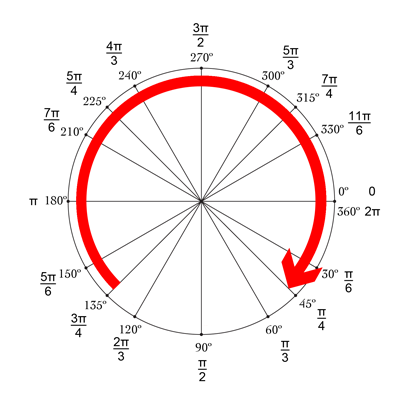kodeco.com Forums

# Core Graphics Tutorial Part 1: Getting Started

In this first part of our Core Graphics tutorial, you'll learn about using Core Graphics to design pixel-perfect views and how to use Xcode's interactive storyboards.

This is a companion discussion topic for the original entry at https://www.raywenderlich.com/411-core-graphics-tutorial-part-1-getting-started

Thanks for the great tutorial! I came up with a slight improvement on the tutorial with regards to the CounterView control, so I thought I’d share and see if others like it.

Instead of adding a separate label to the CounterView control, how about drawing the label in the center of the control so that no extra control is needed?

To accomplish this, I added the following code to the end of the CounterView draw() method, just after drawing the arcs:

// Draw the counter text

// Convert the current value of counter var to a String.
let drawText = String(counter)

// Use the current outlineColor for the color of the counter text. (TODO: even better - make this another public property for text color instead!)
let textColor: UIColor = outlineColor

// Set the font for the counter text (TODO: even better - make this a public property instead!)
let textFont: UIFont = UIFont(name: “AmericanTypewriter”, size: 48)!

// Set up text styling and font attributes
let textStyle = NSAttributedString.TextEffectStyle.letterpressStyle
let textFontAttributes = [
NSAttributedStringKey.font: textFont,
NSAttributedStringKey.foregroundColor: textColor,
NSAttributedStringKey.textEffect : textStyle
] as [NSAttributedStringKey : Any]

// Compute the size of the counter text
let textSize = drawText.size(withAttributes: textFontAttributes)

// Compute the rectangle for drawing the counter text into.
let textRect = CGRect(x: (rect.size.width - textSize.width) / 2, y: (rect.size.height - textSize.height) / 2, width: rect.size.width / 2 + textSize.width / 2, height: rect.size.height - textSize.height)

// Draw the counter text in the center of the control.
drawText.draw(in: textRect, withAttributes: textFontAttributes)

Instead of calling setNeedsDisplay() in the ViewController every time we press the plus/minus buttons, how about putting this into the CounterView control? All we need to do is to add a ‘didSet’ property event to the ‘counter’ property so that every time it gets set, we automatically call setNeedsDisplay() on the control:

``````@IBInspectable var counter: Int = 5 {

didSet {

setNeedsDisplay()
}
}
``````

Hello,

Can someone elaborate on this single line? It’s the only one giving me fits because I’m not sure what angleDifference is meant to represent. Where does 2 * .pi come from?

let angleDifference: CGFloat = 2 * .pi - startAngle + endAngle

I can see that this is then used to divide up the gauge into 8 slices, and I’ve played around with 2 * .pi to see that changing it changes the width of the slice, but I’m still confused. Thanks!

It is due to radians. Degrees and radians do the same job, they measure an angle.

It’s similar to the fact that we have Fahrenheit and Celsius for measuring temperature. Different units measuring the same thing.

So how does this affect what we are doing? A circle is made up of 360 degrees or 2 * Pi radians.

To convert degrees into radians we use the following formula radians = degrees * Pi / 180

If we were to put 360 degrees into that formula we would find that 360 degrees = 2Pi radians.

UIBezierPath takes a startAngle and an endAngle that are both in radians. This means that if we use an angle that is degrees, which is much easier for us to see and understand, it would need to be converted into radians to be used with UIBezierPath.

You can see that here.
let startAngle: CGFloat = 3 * .pi / 4 // This is 135 degrees
let endAngle: CGFloat = .pi / 4 // This is 45 degrees

Using the formula above
startAngleInRadians = 135 * Pi / 180 = 3 * Pi / 4
endAngleInRadians = 45 * Pi / 180 = Pi / 4If we look at this diagram of the unit circle things can become a littler clearer.

We start at 0, on the left hand side. Firstly there are two tracks of numbers. The inner track is in degrees, the outer track is in radians. Going clockwise around the circle, starting from 0. We can see the size of the degrees increases, the radians increase too but that’s a little harder to tell if you are not well versed in fractions.

We are looking to start our arc when we are 135 degrees away from the start of the unit circle and end our arc when we are 45 degrees from the start of the unit circle (the red arrow shows the arc that we want to create)

If we work out the distance that we have travelled, in degrees, from our starting point at 135 degrees to our end point at 45 degrees we get the following:

Firstly we need to calculate the distance from 135 to 360 (as that takes us back to the start of the unit circle, notice how the 0 and 360 are at the same point). So to find that distance we do 360 - 135 = 225 degrees.

Secondly, we need to calculate the distance from the start of the unit circle to its end point at 45 degrees. This is really easy, it’s just 45 degrees .

Finally, the total distance travelled is just 225 + 45 = 270 degrees

We could have done this in one step

angleDifference = 360 - 135 + 45

So the angleDifference is the size of the angle for the arc. Basically how far is it in radians from the startAngle to the endAngle

We can easily convert this to radians (look just before the diagram for the calculation):